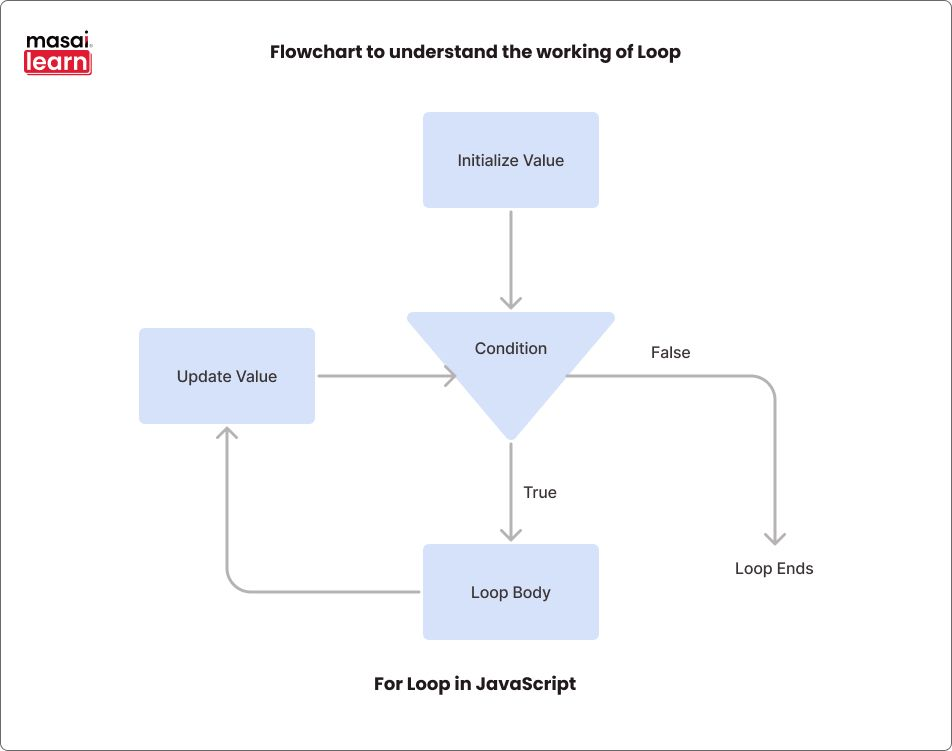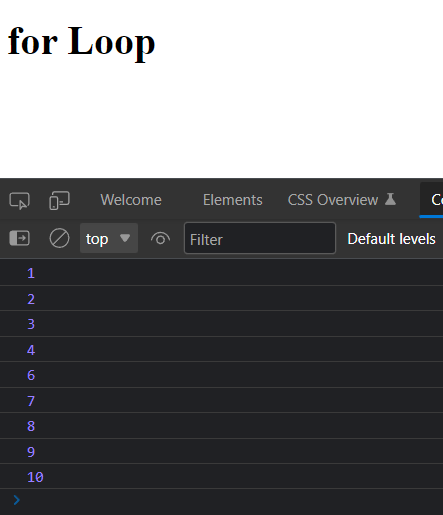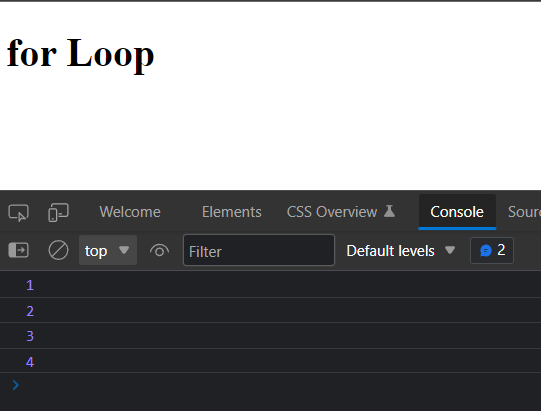All TutorialsCoursesEvents & ContestsModule

For Loop in JavaScript

If Else in JavaScript

For Loop in JavaScript

While Loop in JavaScript

Break and Continue in JavaScript

# For Loop in JavaScript

By Sudip Bhandari

Updated on : 14 Oct 2022

Published on : 14 Oct 2022

## Introduction to Loop

In programming, looping a code means running the code, again and again, multiple times. Loops are used to execute a code block various numbers of times.

Why use a Loop?

To understand the reason behind using a loop, let's see an example. Suppose you want to print a Happy Birthday message 1000 times. In this case, you have to write the code to print Happy Birthday 1000 times which is too time-consuming and tiresome.

However, instead, you can write the code to print the message 1 time and run a loop 1000 times to print it 1000 times.

It will save so much of our time and make our code shorter.

## Different Types of Loop in JavaScript

JavaScript has about 5 types of loops:
• for Loop
• while Loop
• do while Loop
• for of Loop
• for in Loop

## For Loop

The for Loop in JavaScript has 3 expressions:
• Expression 1: to initialize a value
• Expression 2: to check the condition
• Expression 3: to update the value
Syntax:
```.css-135pvw4{margin-top:10px;margin-bottom:10px;width:100%;}```for (initialize value; check condition; update value) {
// code
}
``````
The curly braces, { }, indicate the loop's body which will be executed multiple times.

In the syntax of for loop, if the condition is true, then the body of the loop is executed, and the value is updated. Again, the condition is checked, and if true, the loop body is executed, and the value is updated.## for Loop Example

In the example, we will use a for loop to print Happy Birthday 5 times.
``````<!DOCTYPE html>
<html lang="en">
<meta charset="UTF-8">
<meta http-equiv="X-UA-Compatible" content="IE=edge">
<meta name="viewport" content="width=device-width, initial-scale=1.0">
<title>for Loop</title>

<body>

<h1>for Loop</h1>

<script>
for (let i = 1; i < 6; i++) {
console.log("Happy Birthday");
}
</script>

</body>
</html>
``````

In the above code, Expression 1 initializes the value of i with value 1, Expression 2 checks the condition if the value of i is less than, and Expression 3 updates the value of i by adding 1.

When we run the code, the text Happy Birthday will be printed 5 times. Let's see how it works.

First, the value of i is 1, so the condition i < 6 is true, hence the text is printed, then the value of i is increased to 2. Now the condition is checked again, and it becomes true again, so the text is printed once again. In this way, the text will be printed 5 times from i = 1 to 5.

But when the value of i is 6, the condition i < 6 becomes false, and the body is not executed.

Output:We will see one more example of a for loop, but this time instead of printing the same text, we will print different values.

From the for loop, we know that the update expression updates the value of a variable in every iteration. So, if we print the value of the variable, we will get a different value.

Now let's write a for loop to print numbers from 1 to 10.
``````<!DOCTYPE html>
<html lang="en">
<meta charset="UTF-8">
<meta http-equiv="X-UA-Compatible" content="IE=edge">
<meta name="viewport" content="width=device-width, initial-scale=1.0">
<title>for Loop</title>

<body>
<h1>for Loop</h1>

<script>
for (let i = 1; i < 11; i++) {
console.log(i);
}
</script>

</body>
</html>
``````

In the above code, we are printing the value of i, which is regularly updated by the update expression, i++.

Since the value of i will be different in each iteration, a different value is printed, so we get values from 1 to 10 when we run this code.## Infinite for Loop

When the condition of the for loop is always true, the loop will keep running infinitely. In this case, the loop never ends and is called an infinite for loop.

Let's see one example of the infinite for loop.
``````for (let i = 1; i < 5; i--) {
console.log("Happy Birthday");
}
``````
In this code, the value of i is 1 first, and the condition is i < 5, which is true for the first time. Then the value of i is updated by subtracting 1, so i is 0 now, and the condition is true again.

We are just decreasing the value of i here, so the condition will always be true. Hence, the loop runs infinitely, and Happy Birthday is printed infinite times.

## For Loop with if else

We can also add conditional statements like if else inside a for Loop. Below you can see the syntax of how if else can be used inside the for loop.
``````for (let i = 1; i < 5; i++) {

if (condition) {
// code
} else {
// code
}
}
``````
Here, for every iteration of the for loop, the condition of the if statement is checked. Hence, altogether the condition will be checked 4 times.

Let's understand a scenario where it will be beneficial to use if else inside the for loop. Suppose you are creating a program to print even numbers between 1 to 10. In this case, we can run a for loop from 1 to 10, and in each iteration of the loop, we can check if the number is even or odd.
``````<!DOCTYPE html>
<html lang="en">
<meta charset="UTF-8">
<meta http-equiv="X-UA-Compatible" content="IE=edge">
<meta name="viewport" content="width=device-width, initial-scale=1.0">
<title>for Loop</title>

<body>
<h1>for Loop</h1>

<script>
for (let i = 1; i <= 10; i++) {

// check if the number is even
if (i % 2 == 0) {
console.log(i);
}

// nothing is printed if the condition is false
}
</script>

</body>
</html>
``````
In the above code, we have used an if-else statement inside the for loop. As mentioned earlier, the loop runs 10 times, and in each iteration, we are checking if the number between 1 to 10 is even or not using the condition i % 2 == 0.

If the condition is true, then the number is even and is printed. Otherwise, the number is not printed.

After running the code, your output will look something like this.## For Loop with break Statement

In programming, the break statement is a loop control statement that is used to exit a loop. We will learn about the break statement in more detail in the upcoming article. For now, let's just see how we can use it inside a loop.
``````<!DOCTYPE html>
<html lang="en">
<meta charset="UTF-8">
<meta http-equiv="X-UA-Compatible" content="IE=edge">
<meta name="viewport" content="width=device-width, initial-scale=1.0">
<title>for Loop</title>

<body>
<h1>for Loop</h1>

<script>
for (let i = 1; i <= 10; i++) {

// check if the number is even
if (i == 5) {
break;
}

console.log(i);
}
</script>

</body>
</html>
``````
In the above code, we are using an if statement that checks the condition if the value of i is equal to 5. If this condition is true, then only the break statement is executed.

After running the code, your output will look something like this.From the output, you can see that only values from 1 to 4 are printed, but we have set the for loop to run from 1 to 10. This is happening because when in the fifth iteration, the value of i becomes 5, and the condition i == 5 becomes true. Hence, the break statement is executed, which will terminate the for loop.

We can directly use the break statement with for loop like this,
``````for (let i = 1; i < 10; i++)  {
break;

console.log(i);
}
``````
However, with this, the break statement will directly terminate the loop, and it will be of no use. That's why we usually use the break statement with the conditional statement like if else.

## For Loop with continue Statement

Just like the break, the continue statement also manipulates the normal working of the for loop. However, instead of terminating the loop, it skips the loop execution for that particular iteration.

We will also cover a detailed explanation of the continue statement in the next article. For now, we will just cover the basic introduction.
``````<!DOCTYPE html>
<html lang="en">
<meta charset="UTF-8">
<meta http-equiv="X-UA-Compatible" content="IE=edge">
<meta name="viewport" content="width=device-width, initial-scale=1.0">
<title>for Loop</title>

<body>
<h1>for Loop</h1>

<script>
for (let i = 1; i <= 10; i++) {

// check if the number is even
if (i == 5) {
continue;
}

console.log(i);
}
</script>

</body>
</html>
``````
In the above code, we just changed the break statement to continue. Now, when the value of i is 5, the continue statement will be executed.

After running the code, your output will look something like this.Look carefully, you can see that the value 5 is missing from the output. This is happening because in the fifth iteration, the value of i becomes 5, and the condition i == 5 becomes true.

Hence, the continue statement is executed, which skips the current iteration, meaning that the print statement won't be executed for that iteration. Then the next iteration will be started.

Just like a break, we can also use the continue statement directly with a for loop like this.
``````for (let i = 1; i < 10; i++)  {
continue;

console.log(i);
}
``````
But again, the continue statement will always skip all the iterations, and no value will be printed. That's why we usually use the continue statement with the conditional statement like if else.

## Conclusion

In this article, we learned about the loop and the need for loops in programming. We then learned about the syntax of a for loop with the help of examples. Finally, we covered the working of a for loop with if-else, break, and continue statements.## Check Out Programs Offered By Masai

If you are currently studying in 1st, 2nd or pre-final year of college,

Connect with a growingcommunity of learners